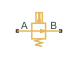# Temperature Control Valve (TL)

Flow control valve with temperature-based actuation

•Libraries:
Simscape / Fluids / Thermal Liquid / Valves & Orifices / Flow Control Valves

## Description

The Temperature Control Valve (TL) block models an orifice with a thermostat as a flow control mechanism. The thermostat contains a temperature sensor and a black-box opening mechanism—one whose geometry and mechanics matter less than its effects. The sensor responds with a slight delay, captured by a first-order time lag, to variations in temperature.

When the sensor reads a temperature in excess of a preset activation value, the opening mechanism is actuated. The valve begins to open or close, depending on the chosen operation mode—the first case corresponding to a normally closed valve and the second to a normally open valve. The change in opening area continues up to the limit of the valve's temperature regulation range, beyond which the opening area is a constant.

A smoothing function allows the valve opening area to change smoothly between the fully closed and fully open positions. The smoothing function does this by removing the abrupt opening area changes at the zero and maximum ball positions.

### Mass Balance

The mass conservation equation in the valve is

`${\stackrel{˙}{m}}_{A}+{\stackrel{˙}{m}}_{B}=0,$`

where:

• ${\stackrel{˙}{m}}_{A}$ is the mass flow rate into the valve through port A.

• ${\stackrel{˙}{m}}_{B}$ is the mass flow rate into the valve through port B.

### Momentum Balance

The momentum conservation equation in the valve is

`${p}_{A}-{p}_{B}=\frac{\stackrel{˙}{m}\sqrt{{\stackrel{˙}{m}}^{2}+{\stackrel{˙}{m}}_{cr}^{2}}}{2{\rho }_{Avg}{C}_{d}^{2}{S}^{2}}\left[1-{\left(\frac{{S}_{R}}{S}\right)}^{2}\right]P{R}_{Loss},$`

where:

• pA and pB are the pressures at port A and port B.

• $\stackrel{˙}{m}$ is the mass flow rate.

• ${\stackrel{˙}{m}}_{cr}$ is the critical mass flow rate:

`${\stackrel{˙}{m}}_{cr}={\mathrm{Re}}_{cr}{\mu }_{Avg}\sqrt{\frac{\pi }{4}{S}_{R}}.$`

• ρAvg is the average liquid density.

• Cd is the discharge coefficient.

• S is the valve inlet area.

• PRLoss is the pressure ratio:

`$P{R}_{Loss}=\frac{\sqrt{1-{\left({S}_{R}/S\right)}^{2}\left(1-{C}_{d}^{2}\right)}-{C}_{d}\left({S}_{R}/S\right)}{\sqrt{1-{\left({S}_{R}/S\right)}^{2}\left(1-{C}_{d}^{2}\right)}+{C}_{d}\left({S}_{R}/S\right)}.$`

### Energy Balance

The energy conservation equation in the valve is

`${\varphi }_{A}+{\varphi }_{B}=0,$`

where:

• ϕA is the energy flow rate into the valve through port A.

• ϕB is the energy flow rate into the valve through port B.

### Valve Opening Area

The valve opening area calculation is based on the linear expression

`${S}_{Linear}=\left(\frac{{S}_{End}-{S}_{Start}}{{T}_{Range}}\right)\left({T}_{Sensor}-{T}_{Activation}\right)+{S}_{Start},$`

where:

• SLinear is the linear valve opening area.

• SStart is the valve opening area at the beginning of the temperature actuation range. This area depends on the Valve operation parameter setting:

• SEnd is the valve opening area at the end of the temperature actuation range. This area depends on the Valve operation parameter setting:

• SMax is the valve opening area in the fully open position.

• SLeak is the valve opening area in the fully closed position. Only leakage flow remains in this position.

• TRange is the temperature regulation range.

• TActivation is the minimum temperature required to operate the valve.

• TSensor is the measured valve temperature.

The valve model accounts for a first-order lag in the measured valve temperature through the differential equation:

`$\frac{d}{dt}\left({T}_{Sensor}\right)=\frac{{T}_{Avg}-{T}_{Sensor}}{\tau },$`

where:

• TAvg is the arithmetic average of the valve port temperatures,

`${T}_{Avg}=\frac{{T}_{A}+{T}_{B}}{2},$`

where TA and TB are the temperatures at ports A and B.

• τ is the Sensor time constant value specified in the block dialog box.

When the valve is in a near-open or near-closed position you can maintain numerical robustness in your simulation by adjusting the parameter. If the parameter is nonzero, the block smoothly saturates the valve area between SLeak and SMax. For more information, see Numerical Smoothing.

## Ports

### Conserving

expand all

Thermal liquid conserving port representing valve inlet A.

Thermal liquid conserving port representing valve inlet B.

## Parameters

expand all

Sign of the change in opening area induced by warming. The opening area can expand with a rise in temperature or it can contract. The change begins at an activation temperature and continues with warming conditions throughout the temperature regulation range of the valve.

The default setting corresponds to a normally closed valve that opens with rising temperature; the alternative setting corresponds to a normally open valve that closes with the same.

Temperature at which the opening mechanism triggers. Warming above this temperature will either open or close the valve, depending on the setting of the Valve operation parameter. The opening area remains variable throughout the temperature regulation range of the valve.

Temperature interval over which the valve opening area varies. The interval begins at the value of the Activation temperature parameter.

Characteristic time for a temperature change to register at the inlet sensor. This parameter determines the delay between the onset of a change and a stable measurement of the change. A value of `0` means that the sensor responds instantaneously to a temperature change.

Opening area of the valve in the fully open position, when the valve is at the upper limit of the pressure regulation range. The block uses this parameter to scale the valve opening throughout the pressure regulation range.

Sum of all gaps when the valve is in the fully closed position. Any area smaller than this value is saturated to the specified leakage area. This contributes to numerical stability by maintaining continuity in the flow.

Continuous smoothing factor that introduces a layer of gradual change to the flow response when the valve is in near-open or near-closed positions.

Areas at the entry and exit ports A and B, which are used in the pressure-flow rate equation that determines the mass flow rate through the orifice.

Correction factor accounting for discharge losses in theoretical flows.

Upper Reynolds number limit for laminar flow through the valve.

## Version History

Introduced in R2016a